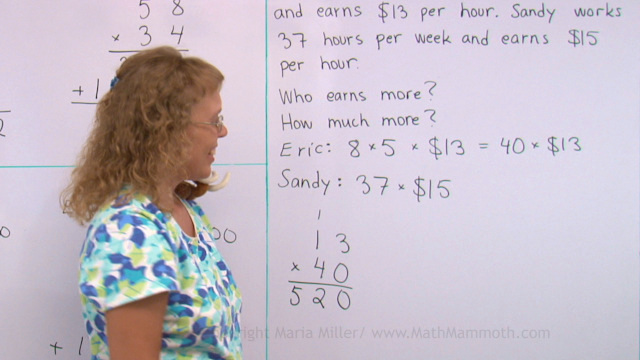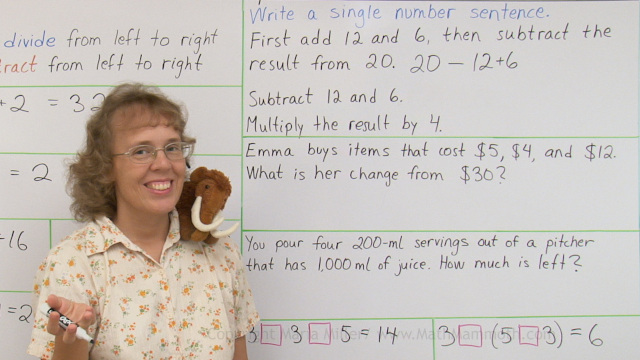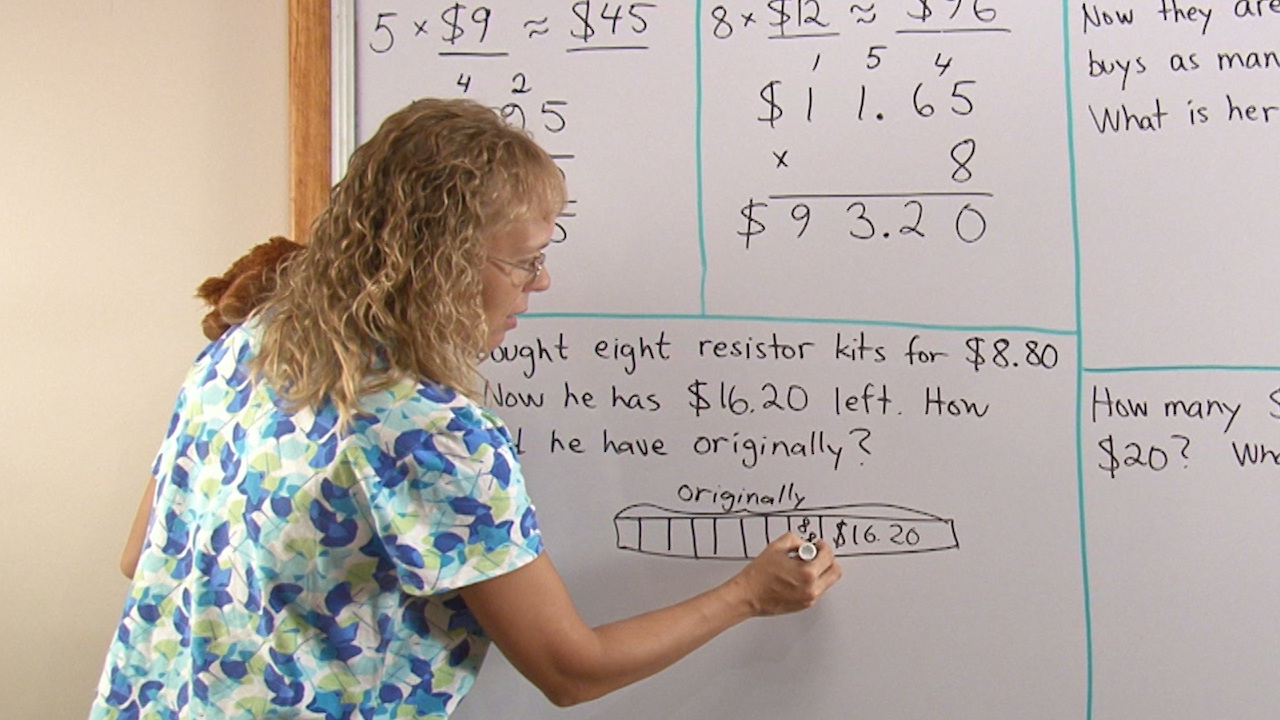Homeschool Buyers Co-op is running a 40% off sale for the large Math Mammoth bundles. The offer expires 8/31/2020.

^

# Free 4th grade math video lessons

This is a collection of free math videos for 4th grade, showing varied exercises for each topic. They match Math Mammoth Grade 4 curriculum but will also work no matter which curriculum you follow (in other words, the videos don't rely on you having Math Mammoth curriculum).

Please choose a topic from the list. Please realize this is not a complete coverage of all 4th grade math, and I hope to add to the collection in the future. Also, some of the videos are older work of mine, but listed here in case they are still helpful to some.

## Addition, subtraction, rounding, and graphsBar models in addition and subtraction problems

Rounding 4-digit numbers

Estimating the final result

The order of operations and writing simple expressions

Bar graph and problems

Round and estimate money amounts

Money word problems

## MultiplicationUse mental math (partial products) to multiply

Multiply 1-digit by 2,3,4-digit number - standard algorithm

Multiplying money amounts: estimation and word problems

Shortcut for 2-digit multiplication when one factor is a multiple of ten

Two-digit multiplication and why we add a zero on the 2nd line

## Measurement

Videos for measurement units are all found on this page. You can also use these shortcut links:

## GeometryVideos for 4th (and 5th) grade geometry are found on the same page. You can also use these shortcut links:

Review: Area and perimeter

Lines, rays, and angles

How to use the protractor

Estimating angles

How to draw a right angle and a rectangle

Parallelograms

Acute, obtuse, and right triangles

## Division and divisibility

Find a fractional part of a quantity (using division)

The order of operations plus writing simple expressions

Basics of division with remainders and practice with it

How to teach long division

Concept of average

Remainders in simple division word problems

What is a factor? A multiple? What does it mean to be "divisible"?

How to find all the factors of a given number

An introduction to prime numbers

## Fractions

Mixed numbers to fractions and vice versa

Adding mixed numbers with like fractional parts

Equivalent fractions

## Decimals

Decimals: tenths

Two decimal digits: hundredths

Back to all videos index

WAIT!

Receive my monthly collection of math tips & resources directly in your inbox — and get a FREE Math Mammoth book!You can unsubscribe at any time.

### Math Mammoth Tour

Confused about the different options? Take a virtual email tour around Math Mammoth! You'll receive:

An initial email to download your GIFT of over 400 free worksheets and sample pages from my books. Six other "TOURSTOP" emails that explain the important things and commonly asked questions concerning Math Mammoth curriculum. (Find out the differences between all these different-colored series!)

This way, you'll have time to digest the information over one or two weeks, plus an opportunity to ask me personally about the curriculum.
A monthly collection of math teaching tips & Math Mammoth updates (unsubscribe any time)### "Mini" Math Teaching Course

This is a little "virtual" 2-week course, where you will receive emails on important topics on teaching math, including:

- How to help a student who is behind
- Troubles with word problems
- Teaching multiplication tables
- Why fractions are so difficult
- The value of mistakes
- Should you use timed tests
- And more!

A GIFT of over 400 free worksheets and sample pages from my books right in the very beginning.A monthly collection of math teaching tips & Math Mammoth updates (unsubscribe any time)
Enter your email to receive math teaching tips, resources, Math Mammoth news & sales, humor, and more! I tend to send out these tips about once monthly, near the beginning of the month, but occasionally you may hear from me twice per month (and sometimes less often).• A GIFT of over 400 free worksheets and sample pages from my books.